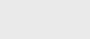3年前 (2020-10-19) |   抢沙发  117

[收起] 文章目录## Java反射代码例子

``````import java.lang.reflect.*;

public class DumpMethods {
public static void main(String args[])
{
try {
Class c = Class.forName(args);
Method m[] = c.getDeclaredMethods();
for (int i = 0; i < m.length; i++)
System.out.println(m[i].toString());
}
catch (Throwable e) {
System.err.println(e);
}
}
}``````

``````public java.lang.Object java.util.Stack.push(
java.lang.Object)
public synchronized
java.lang.Object java.util.Stack.pop()
public synchronized
java.lang.Object java.util.Stack.peek()
public boolean java.util.Stack.empty()
public synchronized
int java.util.Stack.search(java.lang.Object)``````

## 设置为使用反射

`Class c = Class.forName("java.lang.String")` 获取字符串的类对象。另一种方法是使用：`Class c = int.class; or Class c = Integer.TYPE`; 获取基本类型的类信息。后一种方法访问基本类型的包装器的预定义类型字段（例如`Integer`）。

``````Class c = Class.forName("java.lang.String");

Method m[] = c.getDeclaredMethods();

System.out.println(m.toString())``````

## 模拟instanceof操作符

``````class A {}

public class instance1 {
public static void main(String args[])
{
try {
Class cls = Class.forName("A");
boolean b1
= cls.isInstance(new Integer(37));
System.out.println(b1);
boolean b2 = cls.isInstance(new A());
System.out.println(b2);
}
catch (Throwable e) {
System.err.println(e);
}
}
}``````

## 了解类的方法

``````import java.lang.reflect.*;

public class method1 {
private int f1(
Object p, int x) throws NullPointerException
{
if (p == null)
throw new NullPointerException();
return x;
}

public static void main(String args[])
{
try {
Class cls = Class.forName("method1");

Method methlist[]
= cls.getDeclaredMethods();
for (int i = 0; i < methlist.length;
i++) {
Method m = methlist[i];
System.out.println("name
= " + m.getName());
System.out.println("decl class = " +
m.getDeclaringClass());
Class pvec[] = m.getParameterTypes();
for (int j = 0; j < pvec.length; j++)
System.out.println("
param #" + j + " " + pvec[j]);
Class evec[] = m.getExceptionTypes();
for (int j = 0; j < evec.length; j++)
System.out.println("exc #" + j
+ " " + evec[j]);
System.out.println("return type = " +
m.getReturnType());
System.out.println("-----");
}
}
catch (Throwable e) {
System.err.println(e);
}
}
}``````

``````name = f1
decl class = class method1
param #0 class java.lang.Object
param #1 int
exc #0 class java.lang.NullPointerException
return type = int
-----
name = main
decl class = class method1
param #0 class [Ljava.lang.String;
return type = void
-----``````

``````import java.lang.reflect.*;

public class constructor1 {
public constructor1()
{
}

protected constructor1(int i, double d)
{
}

public static void main(String args[])
{
try {
Class cls = Class.forName("constructor1");

Constructor ctorlist[]
= cls.getDeclaredConstructors();
for (int i = 0; i < ctorlist.length; i++) {
Constructor ct = ctorlist[i];
System.out.println("name
= " + ct.getName());
System.out.println("decl class = " +
ct.getDeclaringClass());
Class pvec[] = ct.getParameterTypes();
for (int j = 0; j < pvec.length; j++)
System.out.println("param #"
+ j + " " + pvec[j]);
Class evec[] = ct.getExceptionTypes();
for (int j = 0; j < evec.length; j++)
System.out.println(
"exc #" + j + " " + evec[j]);
System.out.println("-----");
}
}
catch (Throwable e) {
System.err.println(e);
}
}
}``````

``````name = constructor1
decl class = class constructor1
-----
name = constructor1
decl class = class constructor1
param #0 int
param #1 double
-----``````

``````import java.lang.reflect.*;

public class field1 {
private double d;
public static final int i = 37;
String s = "testing";

public static void main(String args[])
{
try {
Class cls = Class.forName("field1");

Field fieldlist[]
= cls.getDeclaredFields();
for (int i
= 0; i < fieldlist.length; i++) {
Field fld = fieldlist[i];
System.out.println("name
= " + fld.getName());
System.out.println("decl class = " +
fld.getDeclaringClass());
System.out.println("type
= " + fld.getType());
int mod = fld.getModifiers();
System.out.println("modifiers = " +
Modifier.toString(mod));
System.out.println("-----");
}
}
catch (Throwable e) {
System.err.println(e);
}
}
}``````

``````name = d
decl class = class field1
type = double
modifiers = private
-----
name = i
decl class = class field1
type = int
modifiers = public static final
-----
name = s
decl class = class field1
type = class java.lang.String
modifiers =
-----``````

## 按名称调用方法

``````import java.lang.reflect.*;

public class method2 {
public int add(int a, int b)
{
return a + b;
}

public static void main(String args[])
{
try {
Class cls = Class.forName("method2");
Class partypes[] = new Class;
partypes = Integer.TYPE;
partypes = Integer.TYPE;
Method meth = cls.getMethod(
method2 methobj = new method2();
Object arglist[] = new Object;
arglist = new Integer(37);
arglist = new Integer(47);
Object retobj
= meth.invoke(methobj, arglist);
Integer retval = (Integer)retobj;
System.out.println(retval.intValue());
}
catch (Throwable e) {
System.err.println(e);
}
}
}``````

`getMethod`用于在类中查找具有两个整型参数类型且具有适当名称的方法。一旦这个方法被找到并捕获到一个方法对象中，它就被调用到一个适当类型的对象实例上。要调用方法，必须构造一个参数列表，基本整数值37和47包装在整数对象中。返回值（84）也被包装在一个整数对象中。

## 创建新对象

``````import java.lang.reflect.*;

public class constructor2 {
public constructor2()
{
}

public constructor2(int a, int b)
{
System.out.println(
"a = " + a + " b = " + b);
}

public static void main(String args[])
{
try {
Class cls = Class.forName("constructor2");
Class partypes[] = new Class;
partypes = Integer.TYPE;
partypes = Integer.TYPE;
Constructor ct
= cls.getConstructor(partypes);
Object arglist[] = new Object;
arglist = new Integer(37);
arglist = new Integer(47);
Object retobj = ct.newInstance(arglist);
}
catch (Throwable e) {
System.err.println(e);
}
}
}``````

## 更改字段值

``````import java.lang.reflect.*;

public class field2 {
public double d;

public static void main(String args[])
{
try {
Class cls = Class.forName("field2");
Field fld = cls.getField("d");
field2 f2obj = new field2();
System.out.println("d = " + f2obj.d);
fld.setDouble(f2obj, 12.34);
System.out.println("d = " + f2obj.d);
}
catch (Throwable e) {
System.err.println(e);
}
}
}``````

## 使用数组

``````import java.lang.reflect.*;

public class array1 {
public static void main(String args[])
{
try {
Class cls = Class.forName(
"java.lang.String");
Object arr = Array.newInstance(cls, 10);
Array.set(arr, 5, "this is a test");
String s = (String)Array.get(arr, 5);
System.out.println(s);
}
catch (Throwable e) {
System.err.println(e);
}
}
}``````

``````import java.lang.reflect.*;

public class array2 {
public static void main(String args[])
{
int dims[] = new int[]{5, 10, 15};
Object arr
= Array.newInstance(Integer.TYPE, dims);

Object arrobj = Array.get(arr, 3);
Class cls =
arrobj.getClass().getComponentType();
System.out.println(cls);
arrobj = Array.get(arrobj, 5);
Array.setInt(arrobj, 10, 37);

int arrcast[][][] = (int[][][])arr;
System.out.println(arrcast);
}
}``````

## Java反射总结

Java反射非常有用，因为它支持按名称动态检索有关类和数据结构的信息，并允许在正在执行的Java程序中对它们进行操作。这个特性非常强大，在其他传统语言中没有等价的东西，如C、C++、FORTRAN或Pascal。### 注册